# How to Construct a Line Parallel to a Given Line Through a Given Point

Parallel lines are lines that are equidistant at all points and would never touch if they went on forever. Sometimes you may be presented with one line and need to create another line parallel to it through a given point. You might be tempted to simply take a straight edge and draw a line that seems right; however, you could not be sure that the line you constructed was technically parallel. Using geometry and a compass, you can plot additional points that will ensure the line you construct is truly parallel.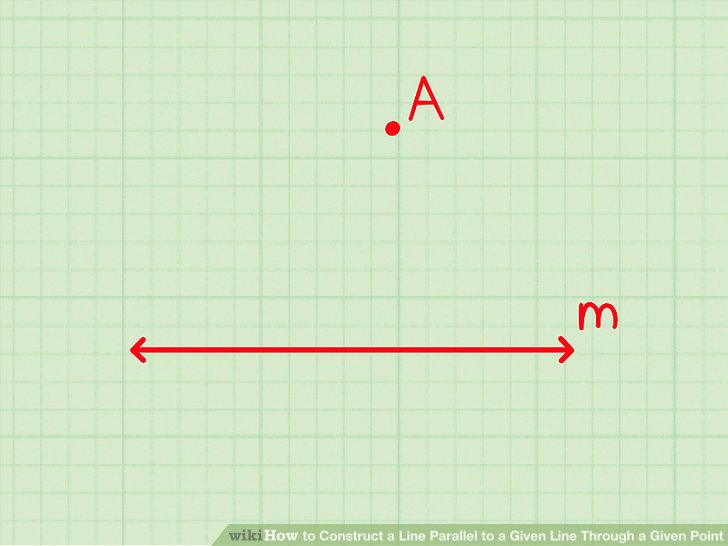# Locate the given line and the given point.

The point will not be on the given line, and can be above or below it. Label the line m{displaystyle m} and the point A{displaystyle A}.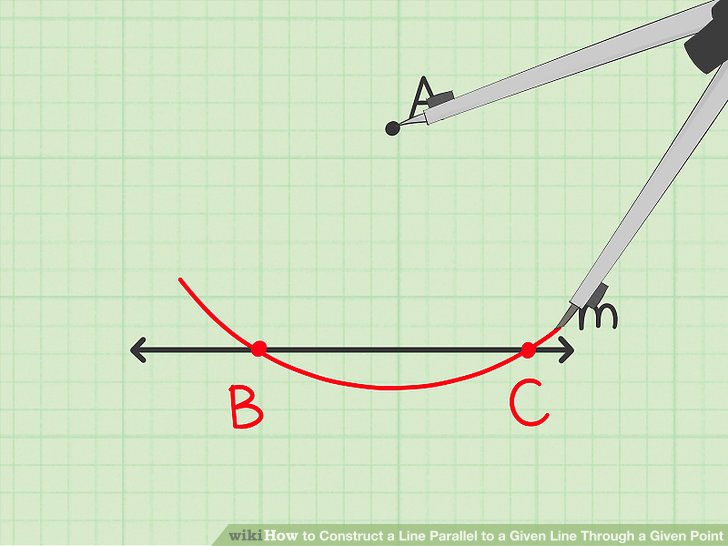# Draw an arc that intersects the given line at two different points.

To do this, place the compass tip on point A{displaystyle A}. Open the compass so that it is wide enough to reach beyond line m{displaystyle m}, then draw an arc that sweeps across the line at points B{displaystyle B} and C{displaystyle C}.# Draw a small arc opposite the given point.

To do this, open the compass a little wider. Set the compass tip on point B{displaystyle B}, and draw an arc that sweeps directly across from point A{displaystyle A}.

If the given point is above the line, you should draw this arc below the line. If the given point is below the line, you should draw this arc above the line.
The arc does not have to be very long, as long as part of it falls directly under the given point.# Draw a another small arc intersecting the previous one.

To do this, keep the compass set to the same width. Set the compass tip on point C{displaystyle C} and draw an arc that intersects the previous small arc. Label this point D{displaystyle D}# Draw a line that connects the given point and the intersection of the two small arcs.

Label this line line n{displaystyle n}. Line n{displaystyle n} is perpendicular to line m{displaystyle m} through points A{displaystyle A} and D{displaystyle D}.
Remember, a perpendicular line is a line that creates a 90 degree angle.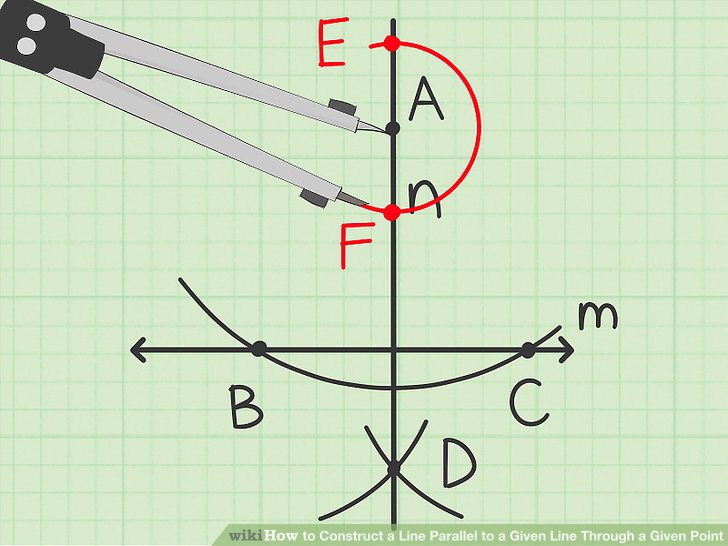# Draw an arc that intersects the perpendicular line at two different points.

To do this, place the compass tip on point A{displaystyle A}, then draw an arc that sweeps across line n{displaystyle n} at points E{displaystyle E} and F{displaystyle F}.# Draw a small arc opposite the given point.

To do this, open the compass a little wider. Set the compass tip on point E{displaystyle E}, and draw an arc that sweeps directly across from point A{displaystyle A}.# Draw a another small arc intersecting the previous one.

To do this, keep the compass set to the same width. Place the compass tip on point F{displaystyle F} and draw an arc that intersects the previous small arc. Label this point G{displaystyle G}.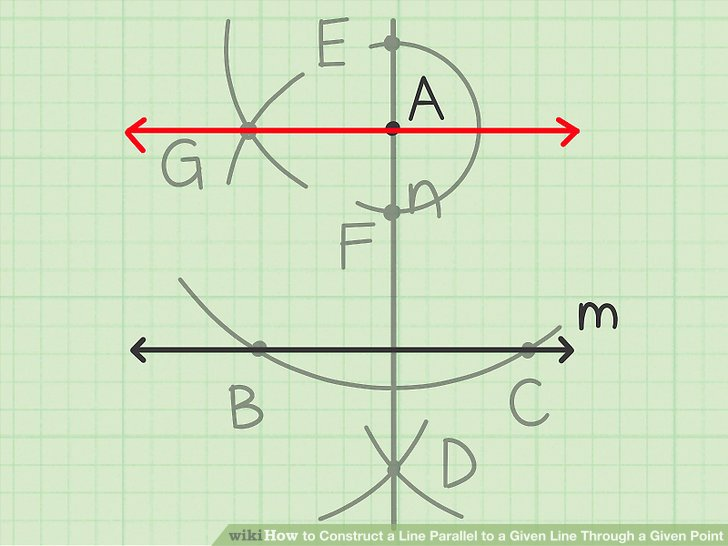# Draw a line connecting the given point to this new point.

This line is perpendicular to line n{displaystyle n}. Thus, it is parallel to line m{displaystyle m} and passes through the given point, point A{displaystyle A}.# Locate the given line and the given point.

The point will not be on the given line, and can be above or below it. Think of this point as one vertex of a rhombus. Since opposite sides of a rhombus are parallel, by drawing a rhombus we can construct a parallel line.

If the line and point are not already labeled, you might want to label them to easily keep track of the steps.
For example, you might have line m{displaystyle m} and point A{displaystyle A}.# Draw the second vertex of the rhombus.

To do this, set the compass tip on the given point and draw an arc that intersects the given line at some point. Do not change the width of the compass.

It does not matter how wide you set the compass, as long as it can intersect the given line.
Make sure the arc reaches above the given point and intersects the given line.
For example, you should set the compass tip at point A{displaystyle A} and create an arc that intersects line m{displaystyle m} at point B{displaystyle B}.# Draw the third vertex of the rhombus.

Using the same compass width, set the compass tip at the second vertex, and draw an arc that intersects the given line at a new point. Do not change the width of the compass.

The arc only has to be long enough to show where it intersects the given line.
For example, you should set the compass tip at point B{displaystyle B} and create an arc that intersects line m{displaystyle m} at point C{displaystyle C}.# Draw the fourth vertex of the rhombus.

Using the same compass width, set the compass at the third vertex, and draw an arc that intersects the first arc you drew (through the second vertex).

The arc only has to be long enough to show where it intersects the first arc.
For example, you should set the compass tip at point C{displaystyle C} and create an arc that intersects the first arc at point D{displaystyle D}.# Draw a line through the first and fourth vertices of the rhombus.

This line will pass through the given point and be parallel to the given line, as the two lines form two opposite sides of a rhombus.
For example, a line drawn through point A{displaystyle A} and point D{displaystyle D} will be parallel to line m{displaystyle m}.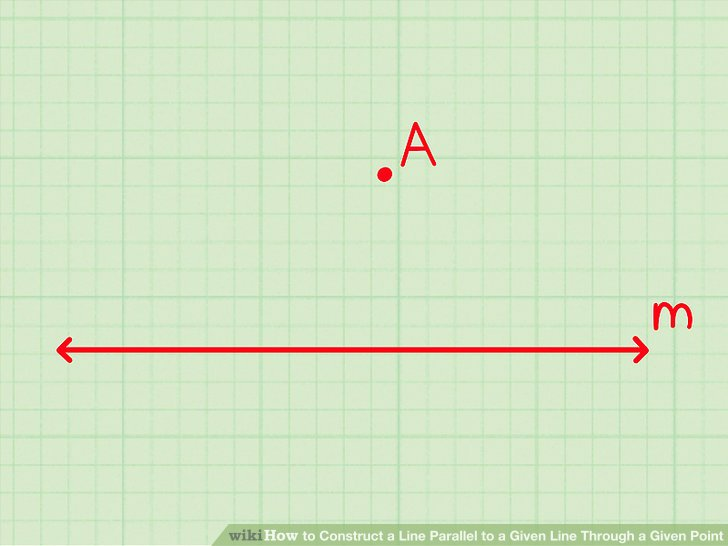# Locate the given line and the given point.

The point will not be on the given line, and can be above or below it.

If the line and point are not already labeled, you might want to label them to easily keep track of the steps.
For example, you might have line m{displaystyle m} and point A{displaystyle A}.# Draw a line through the given point and any point on the given line.

This is the transverse line you will use to draw two corresponding angles, which will help you form the parallel line.

Ensure that the transverse line extends well beyond the given point.
For example, you might draw a line through point A{displaystyle A} that intersects line m{displaystyle m} at point B{displaystyle B}. The new line segment would be AB{displaystyle AB}.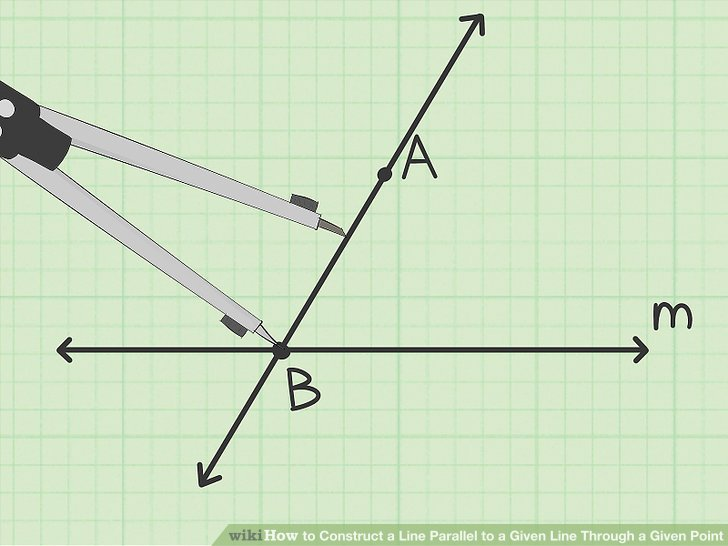# Prepare the compass.

Set the compass to a width that is less than half of the line segment you constructed.

The exact width of the compass does not matter, as long as it is less than half the width of the line segment.
For example, you should set the width of the compass so that it is less than half the width of line segment AB{displaystyle AB}.# Draw the first angle.

Place the tip of the compass on the point where the transverse line intersects the given line. Draw an arc that intersects the transverse line and the given line. Do not change the width of the compass.
For example, you should set the compass at point B{displaystyle B} and draw an arc that intersects line segment AB{displaystyle AB} at point C{displaystyle C} and line m{displaystyle m} at point D{displaystyle D}. This creates the angle CBD{displaystyle CBD}.# Draw an arc.

Using the same compass width, place the tip of the compass on the given point. Draw an arc that intersects the transverse line above the given point, extending to just below the given point.
For example, you should set the compass at point A{displaystyle A} and draw an arc that intersects the transverse line above point A{displaystyle A} at point P{displaystyle P}.# Prepare the compass.

Set the width of the compass to the width of the first angle you created.
For example, the first angle you created was CBD{displaystyle CBD}, so set the tip of your compass on point D{displaystyle D} extend it to point C{displaystyle C}.# Draw the corresponding angle.

Using the width of the first angle, set the tip of the compass at the point on the transverse line above the given point, and draw an arc that intersects the arc you created before.
For example, you should set the compass tip at point P{displaystyle P} and draw an arc that intersects the previous arc at point Q{displaystyle Q}. This gives you angle PAQ{displaystyle PAQ}, which corresponds to angle CBD{displaystyle CBD}.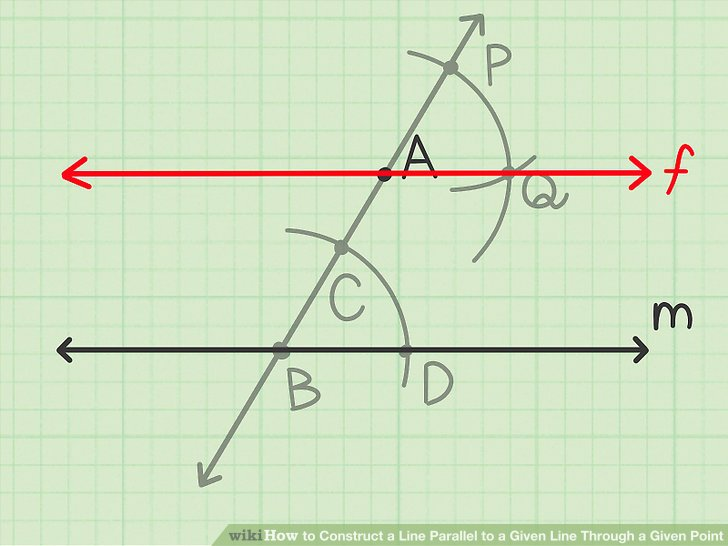# Draw a line through the given point and the point created by the two intersecting arcs.

This line is parallel to the given line through the given point.
For example, a line drawing through point A{displaystyle A} and point Q{displaystyle Q} creates line f{displaystyle f}, which is parallel to line m{displaystyle m}.

Categories:   Youth